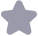### 模拟电路第五版课后习题答案完整版收藏 7

2π T 0 Vs 2 图题1.2.2方波的时域表示 2Y 基波分量 2V4 /R √2 R 2V 三次谐波分量: 2V4 /R T 12 9丌2R (3)3个分量占电阻上总耗散功率的百分比: vs 2V: 2V5 前三者之和为:P3=P+P1+P4F++。2≈0.4758 TT R R 0.475V/R 所占百分比:(P03/P)×100% 100%=95% 7-/2R 1.4放大电路模型 1.4.Ⅰ电压放大电路模型如图题1.4.1(主教材图1.4.2a)所示,设输出 开路电压增益A。=10。试分别计算下列条件下的源电压增益An=/tn 1)R;=10R,R1=10R; (2)R=R,R=R (3)R;=R,/10,R1=R。/10; (4)R;=10R,R1=R。/10 R 解:由图可知,v=(R,+R;), R+R Av;,所以 R (1)R,=10R,R1=10R。时,v。=n(R。+R;) 10 R。+f ×10n,则源电压增益为A2。2 10 100v./11 8.26 1v./10 同理可得 Rs 图题1.4.1电压放大电路模型 (2)A 2.5 2υ 10v./11 (3)An= ≈0.0826 10υ./11 (4)A, ≈0.826 11,/10 1.5放大电路的主要性能指标 1.5.1在某放大电路输人端测量到输入正弦信号电流和电压的峰-峰值 分别为5μA和5mV,输出端接2kΩ电阻负载,测量到正弦电压信号峰-峰 值为1V。试计算该放大电路的电压增益A、电流增益A、功率增益A。,并分 别换算成dB数表示。 1 解:电压增益 200 0.005V 201gA,=201g200≈46dB 电流增益 V/2000g A 100 5x10A 20lg|A2|=201g100≈40dB 功率增益A2=°=(1V)2/20009 20000 ;5×10-3V×5×10-6A 10lgA,=10lg20000≈43dB 1.5.2当负载电阻R1=1kg时,电压放大电路输出电压比负载开路(R1 =)时输出电压减少20%,求该放大电路的输出电阻R。 解:设负载开路时输出电压为v,负载电阻R1=1kΩ时输出电压为υ。, 根据题意 Un=(1-20%)v=0.8v v。vb=R/(R。+Rt1) 则 R。=(v2/v-1)R1=(1/0.8-1)×1×1039=2509 1.5.3一电压放大电路输出端接1kΩ负载电阻时,输出电压为1V,负 载电阻断开时,输出电压上升到1.1V,求该放人电路的输出电阻R。 解:设v为负载电阻断开时的输出电压,即v=1.1V;负载电阻R1= 1kΩ时,输出电压为υ。=1V。根据v。/v=R1/(R。+R1),则输出电阻为 R。=(v/v。-1)R1=(1.1V/1V-1)×1×103.2=1009 1.5.4某放大电路输入电阻R1=10k2,如果用1μA电流源(内阻为) 驱动,放大电路输出短路电流为10mA,开路输出电压为10V。求放大电路接 4k9负载电阻时的电压增益A,、电流增益A1、功率增益A,并分别换算成dB 数表示。 解:根据题意可得输出电阻 R。=10V/(10×10-3A)=1k9 由于放大电路开路输出电压为v=10V,电流信号源电流i。=1μA,负载电阻 R1=4kQ,于是可得 vR1/(R。+R1)10×4×103/(1×103+4×10 800 R 1×10-6×10×103 20lg|A,=20lg800≈58dB t2/(R.+R1)_10/(1×103+4×103) 1×10-6 =2000 201gA1|=20lg2000≈66dB PR1[R/(R。+R1)]2/R i·R 10×4×103/(1×103+4×103)]2/4×10 =1.6×10 1×10°)2×10×10 10lgAn=101g(1.6×10°)≈62dB 1.5.5有以下三种放大电路备用:(1)高输入电阻型:R1=1M9,An =10,Rn1=10k9;(2)高增益型:Ra=10kΩ,An2=100,Ra=1k!; (3)低输出电阻型:R3=10k9,Aa=1,R3=209。用这三种放大电路组 合,设计一个能在1009负载电阻上提供至少0.5W功率的放大器。已知信 号源开路电压为30mV(有效值),内阻为R=0.5M9 解:由于高输入电阻放大电路对电压信号源衰减小,所以输入级(第 级)宜用高输入电阻型放大电路;低输出电阻放大电路带负载能力强,所以 输出级(第三级)宜采用低输出电阻型放大电路;中间级(第二级)用高增益型 于是三种放大电路模型互连组成的放大电路如图解1.5.5所示。 在该放大器中,输入信号源为。=30mV、内阻R,=0.5M2的电压源; 输入级 中间级 输出级 R R R R 十 a Us R, Don Avot Um a 2 Ral lv 13 13 Rely 图解1.5.5 前级放大电路的受控源及输出电阻是后级放大电路的信号源和内阻,而后级放 大电路的输入电阻则是前级放大电路的负载电阻。设三个放大电路模型的输入 电压分别为1、v12和v13,于是 R 1×10 0.03V=0.02V R。+Ri (0.5+1)×10 R 10×10 10×0.02V=0.1Ⅴ R,+R ol 12 (10+10)×10 R 10x10 100 100×0.1V R+R I2 c 2 13 (1+10)×10 R 100 100 000 R+r L 20+100-1 132 放大器输出功率 P。=v0/RL=[(1000/132)2/100]W≈0.574W>0.5W 1.5.6图题1.5.6所示电流放大电路的输出端直接与输人端相连,求输 入电阻R U R R 图题1.5.6电流放大电路 解:根据电路可得 1+i2=1+Bi1=(1+B)h1=(1+B)v,/R1 则 R;=U,/i;=R1/(1+B) 1.5.7在电压放大电路的上限频率点,电压增益比中频区增益下降 3dB,这时在相同输入电压条件下,与中频区比较,输出电压下降到多少? 解:设输入电压为v;中频区输出电压为V。,电压增益为A;上限频 率点输出电压为VH,增益A依题意 201g|Am|-201g|A|=-3dB 又因为 20lg|Am|-20gAo|=20(g|vn/v,|-g|v/v|)=20g 所以 vg|≈0.708|v 在相同输入电压条件下,上限频率点的输出电压约下降到中频区的 0.708。 2运算放大器 2.1集成电路运算放大器 2.1.1电路如图题2.1.1(主教材图2.1.3)所示,运放的开环电压增益 An=10°,输入电阻r1=10°9,输出电阻r。=759,电源电压V,=+10V, V=-10V。(1)求运放输出电压为饱和值时输入电压的最小幅值vp-ny=? (2)输人电流讠1=? 供电 电源 图题2.1.1 解:(1)输人电压的最小幅值φp-n=vo/A,,当υ=±V=±10V时 vr-"N=±10V/10°=±10μV。 (2)输入电流i=(vp-t)/=±10V/10°9=±1×108A。 2.1.2电路如图题2.1.1(主教材图2.1.3)所示,运放的A。=2×103,r 2M,r=759,V+=12V,V=-12V,设输出电压的最大饱和电压值 ±Vm=±11V。(1)如果tp=25μV,v=100μV,试求输出电压vo=?实际 上v应为多少?(2)设Vm=±11V,画出它的传输特性。 解:(1)vo=An(vp-v)=2x103×(25-100)×10-6V=-15V 因为此时运放已饱和,Vm=-1lV,所以vo不能小于-11V,因此v。不 可能达到-15V (2)V=±11V时 11v ±55 2×10 纵轴以v/V取±11V,横轴取(vp-vw)/μV=±55μV,即取a(55μⅤ 11V 11V)、b(-55μV、-11)两点作传输特性,线性区斜率为An=、10-6 =2×103,参见图解2.1.2。 U。N 15 10 11V 55×10°V 2×103 -55μ 100p 55 uv 200 AV 100以 图解2.1 2.3基本线性运放电路 2.3.1设图题2.3.1中的A为理想器件,试求出图a、b、c、d中电路输 出电压v。的值。 解:利用虚短和虚断的概念:Un=",i=0。 由图(a)可知 0 R R 2 0-2Ⅴ2- 0k920k 6 V 由图(b)可得 R 20 R 10 2V=6Ⅴ 1 由图(c)可知 +2V 由图(d)可知 +2V 2 v 10kn 20 kQ 10 kQ 20kQ 2V A 2 R 2y 20k92 20k A A (d) 图题2 2.3.2电路如图题2.3.2所示,设运放是理想的,图a电路中的U,= 6V,图b电路中的v;=10 sin t(mV),图c电路中的vn=0.6V、v2=0.8V 求各放大电路的输出电压v。和a、b图中各支路的电流 解:图(a)利用虚短、虚断的概念:n=n,i=i=0 R 6Ⅴ=2V R1+ R 2+6 R v x2V=4v R3 4=s2-U。(6-2)V~0.33mA R 2k9 R R 0kg2=-0.2mA 4 V =0.8mA R 5 kn i。=i1-i=[0.8-(-0.2)]mA=1mA 图(b)因vn=vn=0,in=i,=0

...展开详情

2019-07-10模拟电路第五版课后习题答案完整版 22积分/C币 立即下载
1/12722积分/C币 立即下载 ＞